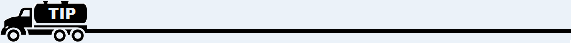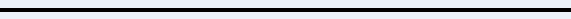﻿ COGS for Propane

# Cost of Goods Sold for Propane

At the end of each period (month), calculate what your Weighted Average Cost is for the total propane sales you sold during the period (month).

To do this, do the following.

1. Multiply your total propane inventory on hand at the first of the month (include bulk, trucks, and yard tanks) times the Weighted Average Cost for last month.

Note: If you don't know this Weighted Average Cost number or are just getting started, use an estimated Weighted Average Cost.

2. For each propane purchase made during the month, multiply the gallons bought times the actual cost per gallon (the amount you paid).

3. Add up the total gallons (beginning inventory gallons plus all gallons purchased during the month).

4. Add up the extended dollar amounts (beginning inventory plus all purchases made during the month).

5. Divide this total extended dollar amount by the extended total gallons.

6. This number is your new Weighted Average Cost per gallon for the month.

7. Multiply this Weighted Average Cost by your total propane sales gallons for the month.

8. This is your total Cost of Goods Sold for propane for the month.

Note: Left-click for an example of how this works.Also, if set up properly, the system will calculate and give you this same number on the Inventory Reconciliation Report.9. When you've finished, print an Income Statement (report GL02 from General Ledger reports). If the COGS account for propane is zero, go to step 10 below. If it is not zero and there is a dollar amount in the COGS account, adjust (subtract) this amount from the Cost Of Goods Sold amount calculated above. Then go to step 10.

10. Key in a Journal Entry for the total Cost of Goods amount as a debit against the appropriate COGS account for propane in your General Ledger and a credit to the corresponding Propane Inventory account.

Note: If you separate your propane costs by category (i.e. Commercial, Residential, and Agricultural, for instance), then you need to repeat this process for each of these categories. You will also need to have a corresponding COGS account and Inventory account for each category as well.For further help regarding Cost Of Goods, go to:
Overview of Cost Of Goods Sold
Cost Of Goods Sold for Large Items
Cost Of Goods Sold for Small Items